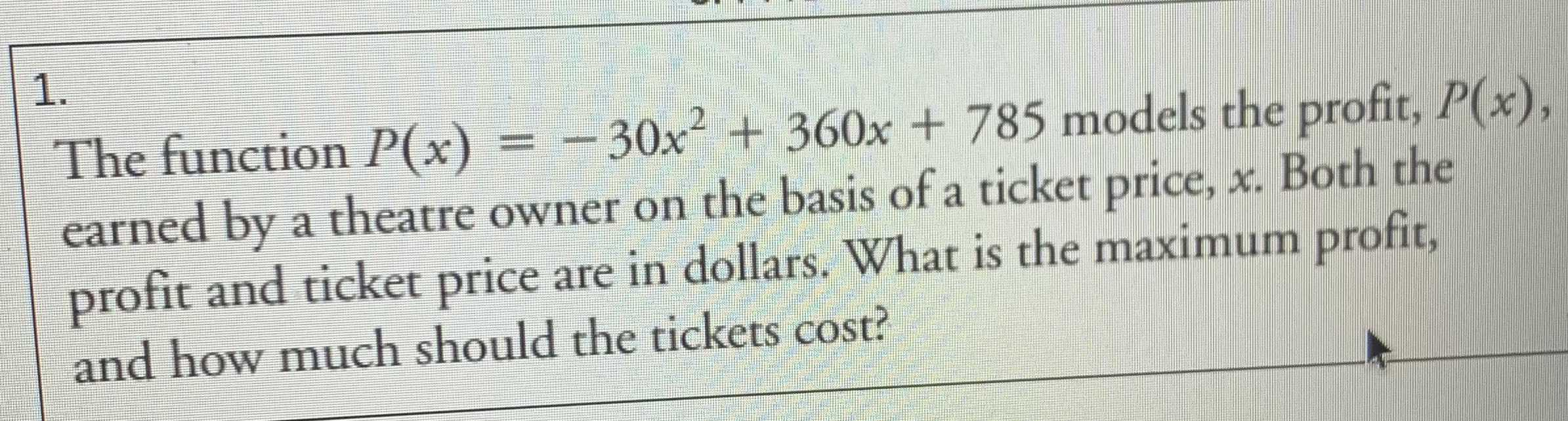### Still have math questions?

Algebra
Question1. The function $$P ( x ) = - 30 x ^ { 2 } + 360 x + 785$$ models the profit, $$P ( x )$$ , earned by a theatre owner on the basis of a ticket price, $$x$$ . Both the profit and ticket price are in dollars. What is the maximum profit, and how much should the tickets cost?